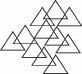# User ForumSubject :IMO    Class : Class 7

Count the number of triangles in the given figure.A22
B20
C19
D21

## Ans 1:

Class : Class 7
first count the small triangles which are 9 and then the big triangles which are 11 so 11+9 =20

## Ans 2:

Class : Class 7
first count the small triangles which are 9 and then the big triangles which are 11 so 11+9 =20